search: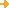advanced search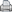printer-friendly version

Title: Between Arithmetic and Algebra
Url: https://www.cut-the-knot.org/ctk/between.shtml
Creator: Bogomolny, Alexander
Publisher: Mathematical Association of America
 Description: What is the difference between the arithmetic 3+5 = 5+3 and the algebraic a+b = b+c? One is a specific fact, another is a pattern valid in a multitude of situations. While arithmetic may hint at some regularities, algebra, as a language, gives expression to acknowledgement of patterns as such. How did people express general ideas before the advent of algebra in the 15th-17th centuries? A discussion of patterns and number systems with a Java applet showing Addition and Multiplication Tables in Various Bases. An interactive column for MAA Online that uses a Java applet to simulate a puzzle or mathematical problem, one not stated directly since the applet is intended to be such that the right answer to an as yet unstated problem should be easy to surmise. Also at http://www.cut-the-knot.com/ctk/between.html.
LC Classification: Science -- Mathematics -- Algebra -- Special topics, A-Z -- Electronic data processing
Science -- Mathematics -- Elementary mathematics. Arithmetic
Science -- Mathematics -- Elementary mathematics. Arithmetic -- Algebra and arithmetic
Science -- Mathematics -- Elementary mathematics. Arithmetic -- Numeration, number concept, numeration systems -- History
Science -- Mathematics -- Elementary mathematics. Arithmetic -- Numeration, number concept, numeration systems -- Number concept (Elementary)
Science -- Mathematics -- Elementary mathematics. Arithmetic -- Study and teaching
Science -- Mathematics -- Elementary mathematics. Arithmetic -- Study and teaching -- Problems, exercises, examinations
Science -- Mathematics -- Tables -- Multiplication tables
GEM Subject: Mathematics
Mathematics -- Technology
Mathematics -- Instructional issues
Mathematics -- Algebra
Mathematics -- Arithmetic
Key Concept: Mathematics -- Algebra
Date Issued: 1998-01
Resource Type: Dataset -- List/Table
Instructional Material
Instructional Material -- Interactive Simulation
Format: Document -- HTML
Application -- Java
Audience: Educator
Learner
Education Level: High School
Higher Education -- Undergraduate (Lower Division)
Higher Education -- Technical Education (Upper Division)
Higher Education -- Technical Education (Lower Division)
Language: English
Rights: Alexander Bogomolny
Access Rights: Free access
 ScreenshotFull Image
Source: Math Forum
Full Record Views: 12
Resource URL Clicks: 10
Cumulative Rating: NOT YET RATED
 Report a Problem with this Resource Record

(no comments available yet for this resource)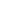forget your password?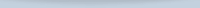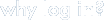Save, organize, and share resources that you find.

Subscribe to bulletins

It's easy, fast, and FREE!get your own loginAMSER =
FREE ONLINE
RESOURCES
for the
CLASSROOM